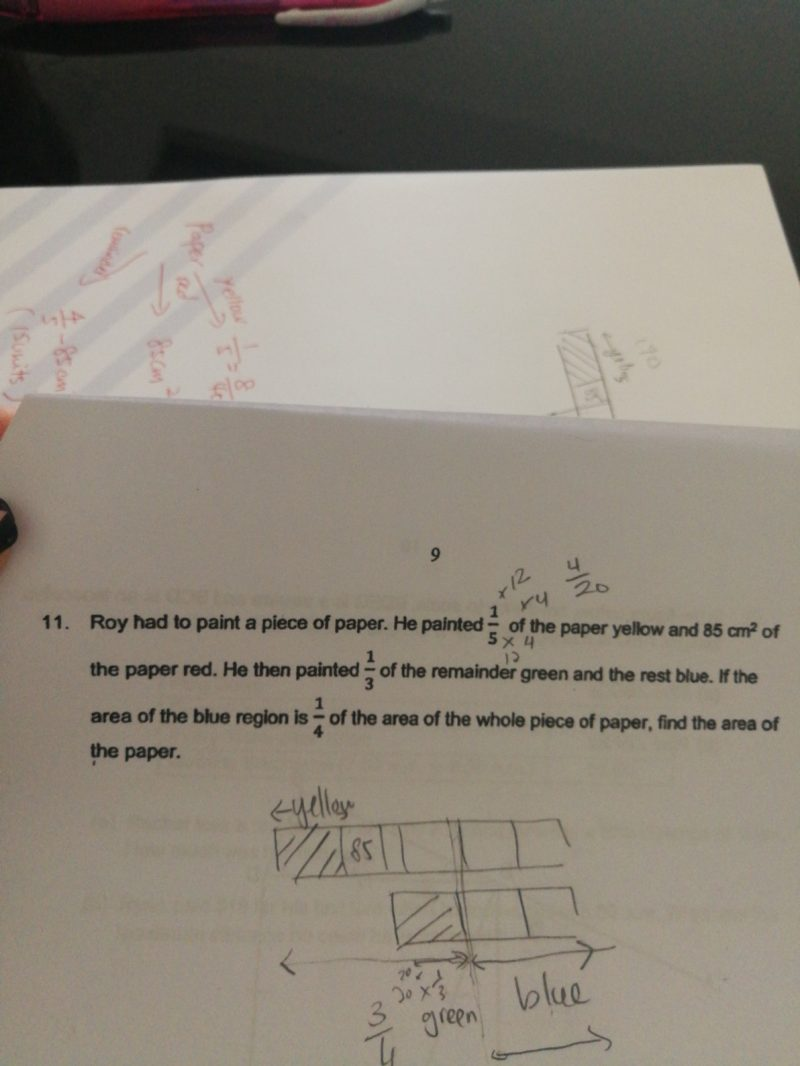# QuestionNeed help!

Thank you.

1 Answer

# Answer

Suggested answer:

Assuming paper is 20 unit (common multiple of 4 and 5)

1/4 x 20u = 5unit

Blue is 2/3 of remaining = 5 unit

Green is 1/3 of remaining = 5 unit/2 = 2.5 unit

Yellow is 1/5 x 20 unit = 4 unit

Red is (20 – 5 -4 -2.5) unit = 8.5 unit

8.5unit = 85cm2

1 unit = 85/8.5 cm2= 10 cm2

10 cmx 20 = 200 cm2

Answer: Paper’s area is 200cm2

0 Replies 0 Likes